# SPC/HW model of water

(Redirected from SPC/HW)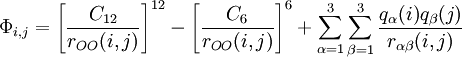$\Phi_{i,j}= \left[ \frac{C_{12}}{r_{OO}(i,j)}\right]^{12} - \left[ \frac{C_{6}}{r_{OO}(i,j)}\right]^{6} + \sum_{\alpha=1}^3 \sum_{\beta=1}^3 \frac{q_\alpha(i) q_\beta(j)}{r_{\alpha \beta}(i,j)}$
 parameter value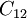$C_{12}$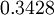$0.3428$ (kJ mol-1) 1/12nm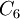$C_6$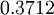$0.3712$ (kJ mol-1) 1/6nm$r_\mathrm{OH}$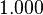$1.000$ Å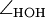$\angle_\mathrm{HOH}$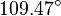$109.47^{\circ}$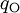$q_{\mathrm{O}}$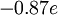$-0.87 e$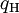$q_{\mathrm{H}}$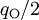$q_{\mathrm{O}}/2$ (charge neutrality)